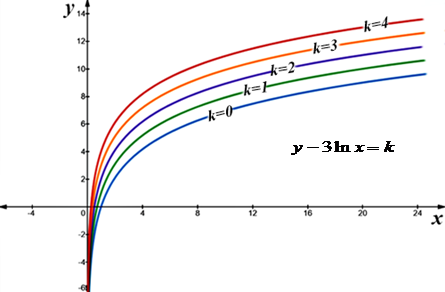# Draw a contour map of the function showing several curves. f(x, y) = y - 3ln(x).

## Question:

Draw a contour map of the function showing several curves.

{eq}f(x, y) = y - 3 \ln(x) {/eq}

## Contour Map:

The surface graphics of the z=f(x,y) functions are usually a bit difficult. It is common for these functions to use level curves called contour lines. If a function is given by the expression z=f(x,y) and we do f(x,y)= k, this equation corresponds to the surface points obtained by sectioning with the plane z=k, parallel to the plane of coordinates z=0. For different k values, different contours form a family of contour lines.

{eq}\eqalign{ & {\text{We can say that a level curves is the set of points in the plane where the function }}z = f\left( {x,y} \right){\text{ }} \cr & {\text{has a constant value }}\,f(x,y) = k{\text{.}} \cr & {\text{Given the function }}\,f\left( {x,y} \right) = z = y - 3\ln x\,\,{\text{ its contours lines are given by:}} \cr & \,\,\,\,\,y - 3\ln x\, = k \cr & {\text{So by placing values to }}\,k{\text{, we get a family of logarithmic curves in the }}xy{\text{ - plane:}} \cr & {\text{ }}\,k = 0\,\, \Rightarrow y - 3\ln x = 0\,\,\, \Rightarrow y = 3\ln x \cr & {\text{ }}\,k = 1\,\, \Rightarrow y - 3\ln x = 1\,\,\, \Rightarrow y = 1 + 3\ln x \cr & {\text{ }}\,k = 2\,\, \Rightarrow y - 3\ln x = 2\,\,\, \Rightarrow y = 2 + 3\ln x \cr & {\text{ }}\,k = 3\,\, \Rightarrow y - 3\ln x = 3\,\,\, \Rightarrow y = 3 + 3\ln x \cr & {\text{ }}\,k = 4\,\, \Rightarrow y - 3\ln x = 4\,\,\, \Rightarrow y = 4 + 3\ln x \cr} {/eq}